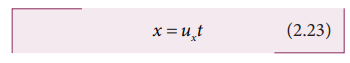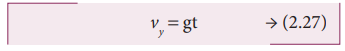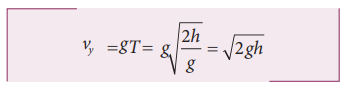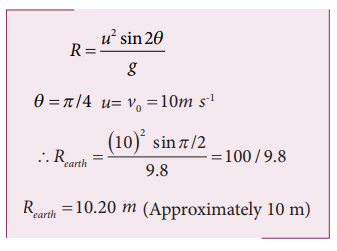Home | | Physics 11th std | Projectile in horizontal projection

# Projectile in horizontal projection

As the ball moves, it covers a horizontal distance due to its uniform horizontal velocity u, and a vertical downward distance because of constant acceleration due to gravity g.

Projectile in horizontal projection

Consider a projectile, say a ball, thrown horizontally with an initial velocityfrom the top of a tower of height h (Figure 2.39).

As the ball moves, it covers a horizontal distance due to its uniform horizontal velocity u, and a vertical downward distance because of constant acceleration due to gravity gThus, under the combined effect the ball moves along the path OPA. The motion is in a 2-dimensional plane. Let the ball take time t to reach the ground at point A, Then the horizontal distance travelled by the ball is x (t) = x, and the vertical distance travelled is y (t) = y

We can apply the kinematic equations along the x direction and y direction separately. Since this is two-dimensional motion, the velocity will have both horizontal component ux and vertical component uy.

## Motion along horizontal direction

The particle has zero acceleration along x direction. So, the initial velocity ux remains constant throughout the motion

The distance traveled by the projectile at a time t is given by the equation x = uxt + 1/2 at2. Since a = 0 along x direction, we have## Motion along downward direction

Here uy = 0 (initial velocity has no downward component), a = g (we choose the +ve y-axis in downward direction), and distance y at time tSubstituting the value of t from equation (2.23) in equation (2.24) we haveEquation (2.25) is the equation of a parabola. Thus, the path followed by the projectile is a parabola (curve OPA in the Figure 2.39).

(1) Time of Flight: The time taken for the projectile to complete its trajectory or time taken by the projectile to hit the ground is called time of flight.

Consider the example of a tower and projectile. Let h be the height of a tower. Let T be the time taken by the projectile to hit the ground, after being thrown horizontally from the tower.

We know that s y = uyt + 1/2 at2 for vertical motion. Here sy = ht = Tuy = 0 (i.e., no initial vertical velocity). ThenThus, the time of flight for projectile motion depends on the height of the tower, but is independent of the horizontal velocity of projection. If one ball falls vertically and another ball is projected horizontally with some velocity, both the balls will reach the bottom at the same time. This is illustrated in the Figure 2.40.(2) Horizontal range: The horizontal distance covered by the projectile from the foot of the tower to the point where the projectile hits the ground is called horizontal range. For horizontal motion, we haveHere, sx = R (range), ux = u, a = 0 (no horizontal acceleration) T is time of flight. Then horizontal range = uT.The above equation implies that the range R is directly proportional to the initial velocity u and inversely proportional to acceleration due to gravity g.

(3) Resultant Velocity (Velocity of projectile at any time): At any instant t, the projectile has velocity components along both x-axis and y-axis. The resultant of these two components gives the velocity of the projectile at that instant t, as shown in Figure 2.41.The velocity component at any t along horizontal (x-axis) is vx = ux + a x t

Since, ux = u, ax = 0 , we getThe component of velocity along vertical direction (y-axis) is v y = uy + a y t

Since, uy = 0, ay = g, we getHence the velocity of the particle at any instant isThe speed of the particle at any instant t is given by(4) Speed of the projectile when it hits the ground: When the projectile hits the ground after initially thrown horizontally from the top of tower of height h, the time of flight isThe horizontal component velocity of the projectile remains the same i.e vx= u

The vertical component velocity of the projectile at time T isThe speed of the particle when it reaches the ground is## Solved Example Problems for Projectile Motion

Example 2.37

Suppose an object is thrown with initial speed 10 m s-1 at an angle π/4 with the horizontal, what is the range covered? Suppose the same object is thrown similarly in the Moon, will there be any change in the range? If yes, what is the change? (The acceleration due to gravity in the Moon gmoon = 1/6 g)

### Solution

In projectile motion, the range of particle is given by,If the same object is thrown in the Moon, the range will increase because in the Moon, the acceleration due to gravity is smaller than g on Earth,The range attained on the Moon is approximately six times that on Earth.

### Example 2.38

In the cricket game, a batsman strikes the ball such that it moves with the speed 30 m s-1  at an angle 30o with the horizontal as shown in the figure. The boundary line of the cricket ground is located at a distance of 75 m from the batsman? Will the ball go for a six? (Neglect the air resistance and take acceleration due to gravity g = 10 m s-2).### Solution

The motion of the cricket ball in air is essentially a projectile motion. As we have already seen, the range (horizontal distance) of the projectile motion is given byThe initial speed30 m s-1

The projection angle θ = 30o

The horizontal distance travelled by the cricket ballThis distance is greater than the distance of the boundary line. Hence the ball will cross this line and go for a six.

Study Material, Lecturing Notes, Assignment, Reference, Wiki description explanation, brief detail
11th Physics : UNIT 2 : Kinematics : Projectile in horizontal projection |